info@biomedres.us   +1 (502) 904-2126   One Westbrook Corporate Center, Suite 300, Westchester, IL 60154, USA   Site Map

Impact Factor : 0.548

# Chemical Spots and Vortices Caused By the Current-Driven Diffusion in Plasma Volume 2 - Issue 2

• AF Ioffe Institute of Physics and Technology, Russia

Received: January 25, 2018;   Published: February 08, 2018

Corresponding author: Vadim Urpin, AF Ioffe Institute of Physics and Technology, Russia

DOI: 10.26717/BJSTR.2018.02.000744

## Abstract

We consider diffusion caused by a combined influence of the electric current and Hall effect, and argue that such diffusion can form in homogeneities of a chemical composition in plasma. Such current-driven diffusion can be accompanied by prop-agation of a particular type of waves in which the impurity number density oscillates alone. These compositional waves exist if the magnetic pressure in plasma is greater than the gas pressure.

Keywords: Plasma magnetic fields; Plasma waves; Plasma chemical spots

## Introduction

Often laboratory and astrophysical plasmas are multicomponent, and diffusion plays an important role in many phenomena in such plasmas. For instance, diffusion can be responsible for the formation of chemical inhomogeneities which influence emission, heat transport, conductivity, etc see, e.g., [1-3]. In fusion experiments, the source of trace elements is usually the chamber walls, and diffusion determines the penetration depth of these elements and their distribution in plasma see, e.g., [4-6]. Even a small admixture of heavy ions increases drastically radiative losses of plasma and changes its thermal properties. In astrophysical conditions, diffusion leads to the formation of element spots detected on the surface of many stars see, e.g., [7-9].

Diffusion in chatged gases or fluids can differ qualitatively from that in media con-sisting of neutral paticles because of the presence of electrons and electric currents. A mean motion of electrons caused by electric currents provides an additional internal force those results in diffusion of trace elements. One more important contribution of electrons in diffusion is relevant to the Hall effect. The magnetic field can magnetize the charged particles that lead to anisotropic transport. In the case of electron transport, such anisotropy is characterized by the Hall parameter, xe = ωBeτe where ωBe = eB /mec is the gyrofrequency of electrons and τe is their relaxation time; B is the magnetic field. In a hydrogen plasma, τe = 3me (kbT )3/2 / 42πe4n∧ see  where n and T are the number density of electrons and their temperature, respectively, A is the Coulomb logarithm. At xe ≥ 1, the rates of diffusion along and across the magnetic field become different and, in general, diffusion can lead to the inhomogeneous distribution of elements.

In this paper, we consider the diffusion process that can lead to formation of chemical inhomogeneities in plasma. This process is caused by a combined influence of electric currents and the Hall effect. Using a simple model, we show that the interaction of the electric current with trace elements leads to their diffusion in the direction perpendicular to both the electric current and magnetic field. This type of diffusion can alter the distribution of chemical elements in plasma and contribute to formation of chemical spots even if the magnetic field is relatively weak and does not magnetize electrons (xe «: 1). We also argue that the current-driven diffusion in combination with the Hall effect can be the reason of the particular type of modes in which the number density of a trace element oscillates alone. The considered diffusive process is rather general and can be important in any medium consisting of charges particles.

## Basic Equations and Diffusion Coefficients

Consider plasma with the magnetic field parallel to the axis z, B = B ~ ez, where (s, ϕ, z) are cylindrical coordinates and ( ~ es,~ eϕ,~ ez) the corresponding unit vectors, respectively. We assume that plasma is cylindrical and the magnetic field depends on the cylindrical radius alone, B = B (s) . Then, the electric current is given by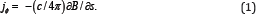We suppose that jϕ → 0 at large s and, hence, B → B0 = const at s → ∞ . Note that the dependence B(s) cannot be an arbitrary function of s because, generally, the cylindrical magnetic configurations are unstable if B(s) increases with s or decreases sufficiently slowly see, e.g., [11-13]. In real conditions, the magnetic field has a more complex topology than our simple model. However, our model describes correctly the main qualitative features of current-driven diffusion and, in some cases; it can even mimic real magnetic fields see, e.g., . We assume that plasma consists of electrons e, protons p, and a small admixture of heavy ions i. The number density of species i am small and it does not influence dynamics of plasma. The partial momentum equations in fully ionized multicom-ponent plasma have been considered by a number of authors see, e.g., . The momentum equation for particles α (α = e, p, i) reads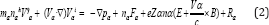The dot denotes the partial time derivative. Here, mα and Zα are the mass and charge number of particles α, nα and pα are their number density and pressure, respectively, Vα is the mean velocity, Fα is an external force acting on the particles α; E and B are the electric and magnetic fields, respectively: Rα is the internal friction force caused by collisions of particles a with other sorts of particles. Since Rα is the internal force, the sum of Rα over a is zero in accordance with Newton’s third law. We neglect the influence of Fα because it is usually small.

If there are no mean hydrodynamic velocity and only diffusive velocities of trace elements are non-vanishing, the partial momentum equation for particles α reads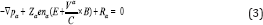The friction forces Ri for trace particles i can be represented as Ri = Rie + Rip where the force Rie is caused by scattering of ions i on electrons and Rip by scattering on protons. If ni « np , Rie is given approximately byWhere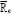is the force acting on the electron gas . In this case,is determined by Scattering of electrons on protons but scattering on ions i gives a small contribution. Therefore we can use forthe expression for  for hydrogen plasma. In our model, this expression readsWhere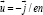is the deference between the mean velocities of electrons and protons α , α , βuT and βuT are coefficients calucated by [15-18] b = B/B. The first two terms on the r.h.s. of Eq. (11) describe the standard friction force caused by a relative motion of the electron and proton gases. The last two terms on the r.h.s. of Eq. (11) represent the so-called thermoforce and are vanishing if ∇T = 0. Taking into account that ~ u = u ~ eϕ in our model and using Coefficients α and α calculated by , we obtain the following expressions for the cylindrical components of Rie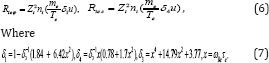The forceconsists of two parts as well,andwhich are proportional to the relative velocity of ions i and protons and to the temperature gradient, respectively. The thermoforce is vanishing in our model. The Friction force is given by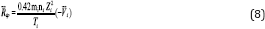See  Where,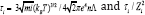is the characteristic timescale of ion-proton scattering; we assume that Coulomb logarithms are the same for all types of scattering. The momentum equation for the species i (see Eq. (3)) contains components of the electric field, Es and Eϕ. These components can be determined from the momentum equations (3) for electrons and protons. Taking into account the condition of hydrostatic equilibrium and quasi-neutrality (nenp), we obtain the following expressions for the radial and azimuthal electric fields.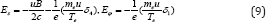Substituting Eqs.(6), (8), and (9) into Eq.(3) for the trace particles i, we arrive to the expression for a diffusion velocity Vi,VnI Are the velocities of ordinary diffusion and VB and Viϕ are the radial and azimuthal diffusion velocities caused by the electric current. The corresponding diffusion coefficients areEqs. (10)-(12) describe the drift of ions i under a combined influence of ∇ni and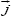. If magnetic field is weak and x « 1, then Eq.(12) yields iWhere c2A = B21(4πnmp) , The Coefficients D is always positive but two other diffusion Coefficients can be positive or negative depending on the parameters of plasma. In the opposite case of a very strong magnetic field, q ≫ 1, Eq.(12) yields.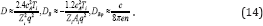In a strong field, diffusion in the radial direction is suppressed because all sorts of particles are magnetized. For instance, the Coefficients D (Eq. (14)) and the corresponding diffusion velocity Vni which characterize the standard diffusion in the s-direction are ≈ q2 » 1 times smaller than those in the case of a weak magnetic field. The Coefficients DB is also approximately q2 times smaller in a strong magnetic field. Note that DB reaches saturation and do not depend on the field strength at q»1. If the electric current is fixed (dB/ds =const), the radial diffusion velocity VB caused by currents decreases ∞ 1/ B. As far as the azimuthal diffusion is concerned, the Coefficients D does not depend on the magnetic field in both cases, strong and weak magnetic fields. However, D in a strong field is greater by a factor.

## Element Spots Caused by Electric Currents

It is generally believed that standard diffusion smoothes chemical inhomogeneities on a diffusion timescale ~ L2 / D where L is the length scale of a non-uniformity. This is not the case, however, for diffusion given by Eq. (12). In this case, chemical inhomogeneities can exist during a much longer time than ~ L2 /D because the equilibrium distribution is reached due to balance of two diffusion processes, standard (∞∇ni) and current-driven ( ∞dB / ds) ones, which push ions in the opposite directions. As a result, Vis = 0 in the equilibrium state and this state can be maintained as long as the electric current exists. Note that the radial velocity is vanishing in the equilibrium state but the azimuthal velocity is non-zero in this state. It turns out that impurities rotate around the magnetic exist even if equilibrium is reached. The direction of rotation depends on the sign of dB/ds and is opposite to the electric current. Since electrons move in the same direction, heavy ions turn out to be carried along the flow of electrons. Different ions move with different velocities around the axis. If the magnetic field is weak (x ≪ 1), the difference between different sorts of ions ∇V is of the order of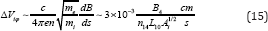Where B4 = B/104G , n14 = n/1014 cm-3, and L10 = L/1010cm . Since different impurities rotate around the magbetic axis with different velocities, periods of such rotation also are different for different ions. The difference in periods can be estimated as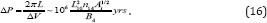If the distribution of impurities is non-axisymmetric then such diffusion in the azimuthal direction should lead to slow variations in the abundance peculiarities. Note that in the case of a strong field (q »1) all sorts of trace particles rotate around the axis with the same period that depends only on the number density and electric current. The condition of hydrostatic equilibrium in our model is given byWhere p and ρ are the pressure and density, respectively. Since the background plasma is hydrogen, p ≈ 2nkBT where kB is the Boltzmann constant? Integrating the s-component of Eq. (13) and taking into account that the temperature is constant in our model, we obtainWhere β= 8πo/B2; (p0, n0, T0, β0) are the values of (p,n,T,β) at S → ∞. Consider the equilibrium distribution of trace elements in cylindrical plasma. In equilibrium distribution, we have Vis =0 and Eq.(10) yields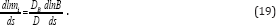The term on the r.h.s. describes the effect of electric currents on the distribution of trace elements. We consider first the case of a weak magnetic field with x ≪ 1. Then, one has from Eq. (17)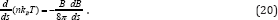Substituting Eq. (19) into Eq.(18) and integrating, we obtain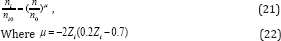And ni0 is the value of ni at s → ∞. Denoting the local abundance of the element i as γi = ni/ n and taking into account Eq. (17), we haveWhere, γ i0 = ni0 /n0 Local abundances turn out to be flexible to the field strength and, particularly, these concerns the ions with large charge numbers. If other mechanisms of diffusion are negligible and the distribution of elements is basically current- driven, then the exponent (μ-1) can reach large negative values for elements with large Zi and, hence, produce strong abundance anormalies. For instance, (μ -1) is equal 1.16, -0.52, and -2.04 for Z i =2, 3, and 4, respectively. Note that (μ - 1) changes its sign as z i increases: (μ-1) > 0 if zi = 2 but (μ-1) < 0 for Zi ≥ 3. Therefore, elements with Zi ≥ 3 are in deficit (γ < γi0) in the region with a weak magnetic field (B < B0) but, on the contrary, these elements should be overabundant in the region where the magnetic field is stronger than B0.

A distribution of the impurities can be substantially different if the magnetic field is strong and q ≫ 1. Using the same procedure as in the case of a weak field, we obtainTherefore, all trace elements with Zi > 1 are overabundant in the regions with the magnetic field weaker than B0. On the contrary, these elements are under abundant in the regions with a stronger magnetic field.

## Compositional waves

In our simplified model of plasma cylinder with the velocity given by Eq.(10), the continuity equation for trace ions i readsConsider the behaviour of small disturbances of the number density of trace ions by making use of a linear analysis of Eq. (25). In the basic (unperturbed) state, plasma is assumed to be in diffusive equilibrium and, hence, the unperturbed impurity number density satisfies Eq. (19). Since the number density of impurity i is small, its influence on parameters of the basic state is negligible. For the sake of simplicity, we consider disturbances that do not depend on z. Denoting disturbances of the impurity number density by δni and linearizing Eq.(25), we obtain the equation governing the evolution of such small disturbances,For the purpose of illustration, we consider only disturbances with wavelengths shorter than the length scale of unperturbed quantities. In this case, we can use the so called local approximation for a consideration of linear waves and assume that small disturbances are ∞ exp (-iks - iMϕ) where k is the wave vector (ks» 1) and M is the azimuthal wave number. Since the basic state does not depend on time, δni can be represented as δni ∞ eiωt-iks-iMϕ where ω should be calculated from the dispersion equation. Substituting δni in such form into Eq. (26), we obtain the following dispersion equation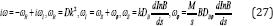This dispersion equation describes spiral waves in which only the number density of impurities oscillates and, therefore, such waves can be called "compositional". The quantity ωR characterizes decay of these waves with the characteristic timescale ~ (Dk2 )-1 typical for a standard diffusion. The frequency ωI describes oscillations of impurities caused by the combined action of electric current and the Hall effect. Note that ωI can be of any sign but ωR is always positive, the frequency ωS characterizes oscillations in the radial direction and ωϕ in the azimuthal direction. The compositional waves are a periodic if ωR > |ωI| and oscillatory if i| > ωR. We consider the compositional waves in particular cases of weak (x « 1) and strong (q»1) magnetic fields. Weak magnetic field (x « 1), If ks » M (radial waves), the condition | ωI |> ωR in a weak field is equivalent toWhereand cs is the sound speed, c 2s = kBT / mp .In the opposite case M » ks (azimuthal waves), the compositional waves are oscillatory if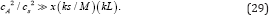Both conditions (28) and (29) require the magnetic field such strong that the magnetic pressure is substantially greater than the gas pressure. The frequency of com-positional waves is higher in the region where the magnetic field has a stronger gradient or, in other words, where the density of electric currents is greater. Note that different impurities oscillate with different frequences. Consider first the radial waves with M = 0. Substituting M = o into Eq. (27), we obtain the dispersion equation for such waves in the formThis dispersion equation describes waves in which only the number density of trace particles oscillates and oscillations of ni occur only in the radial direction. The order of magnitude estimate of ωS yields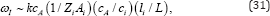Where l i = ciτi i s the mean free-path of ions i. Note that different impurities oscillate with different frequences. Therefore, if there are several sorts of trace ions in plasma, the chemical structure should exhibit variations of local abundances under the influence of compositional waves. The dispersion equation for non- axisymmetric waves with M ≫ ks reads in a weak fieldIn non-axisymmetric waves, trace ions rotate around the cylindrical axis with the frequency ωϕ and decay slowly on the diffusion timescale ~ ωR-1 . The frequency of such waves is typically higher than that of radial waves. One can estimate the ratio of these frequencies asSince these estimates are justified only in the case of a weak magnetic field (x ≪ 1), the period of non-axisymmetric waves is shorter for waves with M > Aix(ks). The ratio of diffusion timescale and period of non-axisymmetric waves is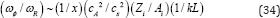And can be large. Therefore, azimuthal waves can be oscillatory as well.

Strong magnetic field (q »1) in a strong magnetic field, the order of magnitude estimates of the characteristic frequences are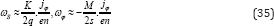Like the case of a weak field, the frequency of compositional waves is higher in the region where the density of electric currents is greater. Oscillations of different trace ions occur with different frequences in radial waves but azinuthal oscillations have the same frequency for different impurities. The frequency of azimuthal waves is higher than that of radial waves if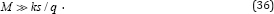If the magnetic field is such strong that q >> 1 then the azimuthal waves oscillate with a higher frequency than the radial ones even for not very large M. The condition that radial waves exist in a strong magnetic field, S| ≫ ωR |, is given by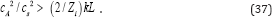Like the case of a weak magnetic field, compositional waves occur in plasma only if the magnetic pressure is greater than the gas one. The analgous condition for azinuthal waves, ω ϕ » ωR , reads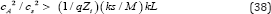Note that this condition can be satisfied even if the magnetic pressure is smaller than the gas one but q and M are large.

## Conclusion

We have considered diffusion of heavy ions under the influence of electric currents. Generally, the diffusion velocity in this case can be comparable to or even greater than that caused by other diffusion mechanisms. The current-driven diffusion can form chemical inhomogeneities even if the magnetic field is relatively weak whereas other diffusion mechanisms require a substantially stronger magnetic field. The current-driven diffusion is relevant to the Hall effect and, therefore, it leads to a drift of ions in the direction perpendicular to both the magnetic field and electric current. As a result, a distribution of chemical elements in plasma depends essentially on the geometry of the magnetic fields and electric current. Chemical inhomogeneities can manifest themselves, for example, by emission in spectral lines and a nonuniform plasma temperature. Usually, diffusion processes play an important role in plasma if hydrodynamic motions are very slow. In some cases, however, chemical spots can be formed even in flows with a relatively large velocity but with some particular topology (for example, a rotating flow).

Our study reveals that a particular type of waves may exist in multicomponent plasma in the presence of electric currents. These waves are slowly decaying and characterized by oscillations of the impurity number density alone. They exist only if the magnetic field is such strong that the magnetic pressure is greater than the gas pressure. Generally, the frequency of such waves turns out to be different for different impurities. This frequency is rather low and is determined mainly by a diffusion timescale. If M = 0, it can be estimated as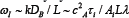where λ = 2π/ k is the wavelength of waves. In astrophysical conditions, such waves can manifest themselves in the atmospheres of magnetic stars where the magnetic field is of the order of 104 G and the number density and temperature are 1014 cm-3 and 104 K, respectively. If the length scale, L, and the wavelength, λ, are of the same order of magnitude (for in-stance, cm), then the period of compositional waves is yrs. This is much shorter than the stellar lifetime (see, e.g., [17, 18]) and generation of such waves in the atmospheres should lead to spectral variability with the corresponding timescale.

Compositional waves can occur in laboratory plasmas as well but their frequency is essentially higher. If B~ 105G, n~ 1015cm 3 , T~ 106K , and L~λ~102 cm, then the period of compositional waves is ~ 10-8 s . Note that this is only the order of magnitude estimate but frequencies of various impurities can differ essentially since the period of compositional waves depend on the sort of heavy ions. In terrestrial conditions, the compositional waves also can manifest themselves by oscillations in spectra. Note that these waves exist only if the magnetic pressure is greater than the gas pressure. The current-driven diffusion can be important not only in plasma but in some conductive fluids if the magnetic field is sufficiently strong there.